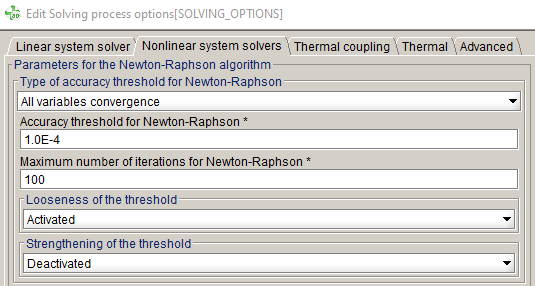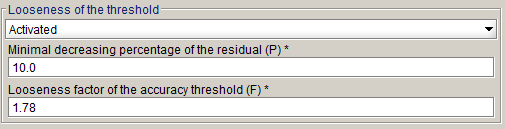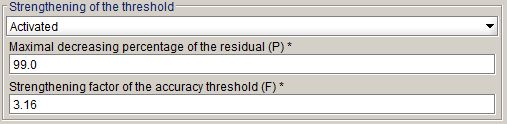# Adjusting parameters in the Newton-Raphson method

Newton-Raphson parameters and strategies are set automatically to ensure robustness and fast non-linear solving. It is nevertheless possible for the user to adjust these parameters, using the Nonlinear system solvers tab of the Solving process options box.

The user can adjust the following Newton-Raphson parameters:• Type of accuracy threshold: three options are available, namely:
• All variables convergence: all variables of the system must satisfy the required precision;
• Global convergence: the variable exhibiting the hardest convergence must satisfy the required precision;
• Automatic.
The Automatic option corresponds to All variables convergence in all cases, with a few remarkable exceptions, which are listed below:
• projects containing hysteretical materials (in 2D and 3D);
• when the optimal method with a stabilization stage relaxation method is used;
• in the case of 2D initialization for Skew.

For all these exceptions, the Automatic option corresponds to Global convergence instead, since this approach leads to better performance and improved robustness.

• The threshold: relative epsilon value below which Newton-Raphson non-linear solver stops. Depending on the activation of looseness and/or strengthening, this threshold is fixed or is the starting point of the adaptive criteria strategy. Default value is 10e-4.
• Maximum number of Newton-Raphson iterations: the default value is 100.
• Adaptive criteria strategy relies on hard or easy convergence detection.
• Looseness of the threshold: this adaptive criteria strategy is meant to ease hard convergence. The adaptation is based on the residual decrease between two consecutive Newton-Raphson iterations. If the decrease between these two residuals is less than a percentage P (default is 10%), we consider a hard convergence situation (Red region on Figure 1). If we have a hard convergence situation, the threshold is multiplied by a factor F (default is 1.78) to ease the convergence.

Percentage P and factor F can be modified in advanced mode:This strategy is activated by default, except:
• for projects containing hysteretical materials (in 2D and 3D);
• when the optimal method with a stabilization stage relaxation method is used;
• in the case of 2D initialization for Skew;
• in 2D projects containing materials with the magneto-mechanical property B(Stress).
• Strengthening of the threshold : this adaptive criteria strategy is meant to harden easy convergence. The adaptation is based on the residual decrease between two consecutive Newton-Raphson iterations.

If the decrease between these two residuals is more than a percentage P (default is 99%), we consider a too easy convergence situation (Yellow region on Figure 1). If we have too easy convergence situation, the threshold is divided by a factor F (default is 3.16) to harden the convergence.

Percentage P and factor F can be modified in advanced mode:This strategy is activated by default, except:
• for projects containing hysteretical materials (in 2D and 3D);
• when the optimal method with a stabilization stage relaxation method is used;
• in the case of 2D initialization for Skew;
• in 2D projects containing materials with the magneto-mechanical property B(Stress).

## Under-relaxation method (3D)

There are five methods for defining the relaxation coefficient a of the Newton-Raphson method:

• the stairs method, where the coefficient is determined at each Newton-Raphson iteration, according to a simple law that depends on the obtained accuracy by the Newton-Raphson algorithm,
• the fixed method, where the user inserts the coefficient value which is thus constant throughout the solving process,
• the optimal method, where the coefficient is determined at each Newton-Raphson iteration by minimizing the residual of the nonlinear system to be solved,
• the automatic method, where the program automatically determines, depending on the formulation, which method is used, the "stairs" or the "optimal" one.
• The maximal factor method, which computes at each Newton-Raphson iteration the largest coefficient such that the residual of the non-linear system to be solved decreases.

The automatic method is the method chosen by default. These four methods are detailed in the following paragraph.

## Stairs method

The relaxation coefficient is determined at each Newton-Raphson iteration, function of the value of the precision εi-1 obtained at the previous iteration, following the variation law given below:

• at the first iteration:

α = 0,5

• at the next iterations:
• if εi-1 ∈ [0,1 ; +∞[ : α = 0,5
• if εi-1 ∈ [0,01 ; 0,1[ : α = 0,75
• if εi-1 ∈ [0 ; 0,01[ : α = 1

## Fixed method

The value of the relaxation coefficient is inserted by the user in the interval ]0 ; 1]. The value of this coefficient is thus constant throughout the solving process.

## Optimal method

The relaxation coefficient is determined at each iteration. The principle of the method consists of computing this coefficient so that the following objective function is minimum:

${W}^{i}=\sum _{j=1}^{n}{\left({R}_{j}^{i}\right)}^{2}$

where ${R}_{j}^{i}$ is the j-th component of the residual R(Xi) at the i-th current Newton-Raphson iteration (R(Xi) is a vector with n elements). n is the number of unknowns of the system.

The search of the objective function minimum is carried out using an iterative method, due to the fact that W cannot be explicitly expressed function of α. The search is performed by computing the values of Wi for the values of

α = αk = 1/2k-1 (α = 1 then 1/2, 1/4, 1/8...), and it is stopped when Wik+1 at the iteration k+1 is greater than Wik at the previous iteration k. Indeed, during the iterations, α = αk = 1/2k-1 decreases and Wik decreases up to a certain iteration and then it increases.

## Automatic method

Flux 3D automatically determines based on the formulations used for the regions, which is the best relaxation method.

Flux 3D automatically chooses the optimal method, except for the regions that use a surface impedance formulation in magnetic scalar potential (reduced or total). In that case, the stairs method will be used.

In Flux 2D, for projects containing materials with the magneto-mechanical property B(Stress), the optimal method is selected by the automatic method.

## Maximal factor method

The relaxation coefficient (α) is computed at each Newton-Raphson iteration. This method consists in searching the largest coefficient satisfying a decrease of the residual of the non-linear system. To do that, the residual is computed with different values of α, by first trying to increase this coefficient (maximum to 1) before decrease it only if the residual of the non-linear system did not decrease with respect to the previous Newton-Raphson iteration.

More in details, the strategy is the following: at the ith Newton-Raphson iteration, the algorithm starts with the relaxation coefficient α of the previous Newton-Raphson iteration (α=1 at the first iteration). Then it first increases that coefficient (α = min(αφ,1), with φ the golden ratio) and computes the new residual of the non-linear system.

• If that residual is less than the residual of the previous Newton-Raphson iteration, the algorithm continues to increase that coefficient with the same rule, until it reaches α = 1 or obtains a residual greater than the one of the previous Newton-Raphson iteration. It will then keep the last α (the largest) which decreases the residual.
• If this residual is greater than the residual of the previous Newton-Raphson iteration, the algorithm decreases that coefficient (α = max(α/φ,β), with β the minimum relaxation coefficient, depending on the project and defined by Flux). If the residual becomes less than the one of the previous Newton-Raphson iteration then the algorithm keeps that coefficient, else it continues to decrease the coefficient, following the same rule.